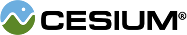#Plane

#### new Cesium.Plane(normal, distance) Core/Plane.js 37

A plane in Hessian Normal Form defined by
```ax + by + cz + d = 0
```
where (a, b, c) is the plane's `normal`, d is the signed `distance` to the plane, and (x, y, z) is any point on the plane.
Name Type Description
`normal` Cartesian3 The plane's normal (normalized).
`distance` Number The shortest distance from the origin to the plane. The sign of `distance` determines which side of the plane the origin is on. If `distance` is positive, the origin is in the half-space in the direction of the normal; if negative, the origin is in the half-space opposite to the normal; if zero, the plane passes through the origin.
##### Example:
``````// The plane x=0
var plane = new Cesium.Plane(Cesium.Cartesian3.UNIT_X, 0.0);``````

### Members

#### staticconstantCesium.Plane.ORIGIN_XY_PLANE : Plane Core/Plane.js 157

A constant initialized to the XY plane passing through the origin, with normal in positive Z.

#### staticconstantCesium.Plane.ORIGIN_YZ_PLANE : Plane Core/Plane.js 165

A constant initialized to the YZ plane passing through the origin, with normal in positive X.

#### staticconstantCesium.Plane.ORIGIN_ZX_PLANE : Plane Core/Plane.js 173

A constant initialized to the ZX plane passing through the origin, with normal in positive Y.

#### distance : Number Core/Plane.js 63

The shortest distance from the origin to the plane. The sign of `distance` determines which side of the plane the origin is on. If `distance` is positive, the origin is in the half-space in the direction of the normal; if negative, the origin is in the half-space opposite to the normal; if zero, the plane passes through the origin.

#### normal : Cartesian3 Core/Plane.js 52

The plane's normal.

### Methods

#### staticCesium.Plane.fromCartesian4(coefficients, result) → Plane Core/Plane.js 108

Creates a plane from the general equation
Name Type Description
`coefficients` Cartesian4 The plane's normal (normalized).
`result` Plane optional The object onto which to store the result.
##### Returns:
A new plane instance or the modified result parameter.

#### staticCesium.Plane.fromPointNormal(point, normal, result) → Plane Core/Plane.js 79

Creates a plane from a normal and a point on the plane.
Name Type Description
`point` Cartesian3 The point on the plane.
`normal` Cartesian3 The plane's normal (normalized).
`result` Plane optional The object onto which to store the result.
##### Returns:
A new plane instance or the modified result parameter.
##### Example:
``````var point = Cesium.Cartesian3.fromDegrees(-72.0, 40.0);
var normal = ellipsoid.geodeticSurfaceNormal(point);
var tangentPlane = Cesium.Plane.fromPointNormal(point, normal);``````

#### staticCesium.Plane.getPointDistance(plane, point) → Number Core/Plane.js 138

Computes the signed shortest distance of a point to a plane. The sign of the distance determines which side of the plane the point is on. If the distance is positive, the point is in the half-space in the direction of the normal; if negative, the point is in the half-space opposite to the normal; if zero, the plane passes through the point.
Name Type Description
`plane` Plane The plane.
`point` Cartesian3 The point.
##### Returns:
The signed shortest distance of the point to the plane.